Test: Signals & Systems - 1

# Test: Signals & Systems - 1

Test Description

## 15 Questions MCQ Test GATE ECE (Electronics) 2023 Mock Test Series | Test: Signals & Systems - 1

Test: Signals & Systems - 1 for Electronics and Communication Engineering (ECE) 2023 is part of GATE ECE (Electronics) 2023 Mock Test Series preparation. The Test: Signals & Systems - 1 questions and answers have been prepared according to the Electronics and Communication Engineering (ECE) exam syllabus.The Test: Signals & Systems - 1 MCQs are made for Electronics and Communication Engineering (ECE) 2023 Exam. Find important definitions, questions, notes, meanings, examples, exercises, MCQs and online tests for Test: Signals & Systems - 1 below.
Solutions of Test: Signals & Systems - 1 questions in English are available as part of our GATE ECE (Electronics) 2023 Mock Test Series for Electronics and Communication Engineering (ECE) & Test: Signals & Systems - 1 solutions in Hindi for GATE ECE (Electronics) 2023 Mock Test Series course. Download more important topics, notes, lectures and mock test series for Electronics and Communication Engineering (ECE) Exam by signing up for free. Attempt Test: Signals & Systems - 1 | 15 questions in 45 minutes | Mock test for Electronics and Communication Engineering (ECE) preparation | Free important questions MCQ to study GATE ECE (Electronics) 2023 Mock Test Series for Electronics and Communication Engineering (ECE) Exam | Download free PDF with solutions
 1 Crore+ students have signed up on EduRev. Have you?
Test: Signals & Systems - 1 - Question 1

### If a real function f(t) has Fourier transform F(ω), then Fourier transform of f(t) - f(-t) will be

Detailed Solution for Test: Signals & Systems - 1 - Question 1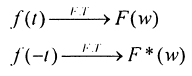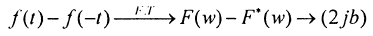If F(w)is a complex no.a+jb

then Ft (w) is (a-jb)

SoF.T.of f(t)-f(-t) will bean imaginary number equal to (2 jb)

Test: Signals & Systems - 1 - Question 2

### If impulse response of a discrete time LTI system is an u[n] then its step response is

Detailed Solution for Test: Signals & Systems - 1 - Question 2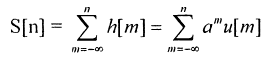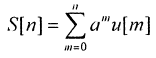Test: Signals & Systems - 1 - Question 3

### Q. If transfer function H(s) of a system is given as H(s) =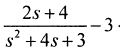< Re {s} < -1 then value of h(t) is given as.

Detailed Solution for Test: Signals & Systems - 1 - Question 3

Take partial fraction and then take inverse according to range of ROC.

Test: Signals & Systems - 1 - Question 4

if u(t) is unit step and δ(t) is unit impulse function then Inverse z, Transform of F(z) = 1/z + 1For k > 0 is

Test: Signals & Systems - 1 - Question 5

What is the value of Fundamental period of sequence x[n] = 3 sin (1.3 nπ+ π/2) + sin (1.2π)

Test: Signals & Systems - 1 - Question 6

If Fourier transform of x[n] is X(e'°') then Fourier transform of (- 1)n x[n] will be

Detailed Solution for Test: Signals & Systems - 1 - Question 6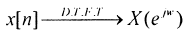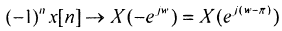Test: Signals & Systems - 1 - Question 7

A continuous time system is described by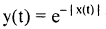where y(t) is output and x(t) is input, then y(t)is bounded.

Detailed Solution for Test: Signals & Systems - 1 - Question 7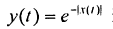if x (t) is not bounded then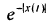is bounded.

Test: Signals & Systems - 1 - Question 8

Magnitude of Fourier transform x(t) & y(t) are shown in figure. If phases of X(ω) and Y(ω) are zero for all w. The magnitude and frequency units are identical in both figure then relation between y(t) and x(t) is expressed as :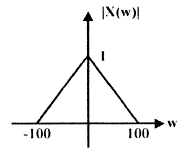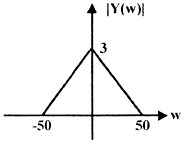Test: Signals & Systems - 1 - Question 9

X (z) =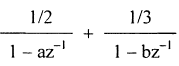, if lal & Ibl < 1 with ROC, specified as

|a| < |z| < |b| then x  of corresponding sequence is given by.

Detailed Solution for Test: Signals & Systems - 1 - Question 9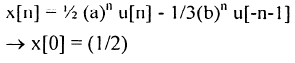Test: Signals & Systems - 1 - Question 10

If impulse response of a system is h(t) = e-|t|then.

Detailed Solution for Test: Signals & Systems - 1 - Question 10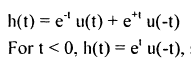so it is a non - causal system.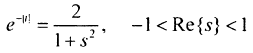So         it is a stable - system.

Test: Signals & Systems - 1 - Question 11

A box has 4 white and 3 red balls. Two balls are taken out in succession. What is the probability that both are white?

Test: Signals & Systems - 1 - Question 12

For a given discrete time sequencex[n] = 2, 0, - 1, - 3, 4, 1, - 1 ) then value of X (e) is

Test: Signals & Systems - 1 - Question 13

Which of the following transfer function corresponds to an unstable system

Test: Signals & Systems - 1 - Question 14

14 If average power and energy of signal u(t) are P and E then

Detailed Solution for Test: Signals & Systems - 1 - Question 14

Just use formula for energy and power.

Test: Signals & Systems - 1 - Question 15

The value of δ(at) and δ(an) are respectively.

Detailed Solution for Test: Signals & Systems - 1 - Question 15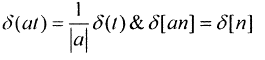## GATE ECE (Electronics) 2023 Mock Test Series

21 docs|263 tests
Information about Test: Signals & Systems - 1 Page
In this test you can find the Exam questions for Test: Signals & Systems - 1 solved & explained in the simplest way possible. Besides giving Questions and answers for Test: Signals & Systems - 1, EduRev gives you an ample number of Online tests for practice

## GATE ECE (Electronics) 2023 Mock Test Series

21 docs|263 tests(Scan QR code)